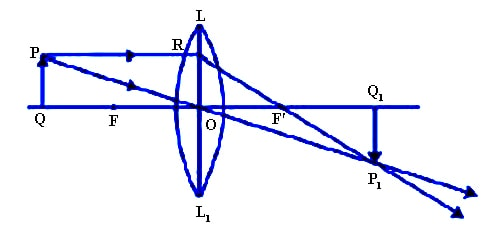Physics

# Explain Image of Extended object of Convex Lens

Suppose LOL1 is a convex lens. FOF′ is its principal axis, O is its optical center and F is its principal focus. An extended object PQ is placed perpendicularly on the principal axis in front of the lens at a point between f and 21 The image of the object PQ is to be drawn.

A ray of light PR coming from P parallel to the principal axis incidents at R and is refracted through the principal focus F along the path RF′P1. Another ray PO from P passes through the optical center O and is refracted along the path RF′P1. The refracted rays RF′P1 and OP1 meet at point P1. So, P1 is the real image of point P. From point P1 a perpendicular P1 Q1 is drawn on the principal axis. So, P1 Q1 is the real image of PQ. Here OQ is the distance of the object and OQ1 is the distance of the image.In this case, the image is real, inverted and magnified.

Depending on the position of the object on the principal axis the nature of image may be real, virtual, erect, inverted, magnified, diminished or equal in size to the object.

If the position of the object is between the focus and optical center the image will be virtual, erect and magnified.